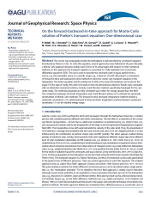The Heliospheric Modulation Model describes the propagation of Galactic Cosmic Rays into the heliosphere from Termination Shock (the outer border) down to Earth orbit. The Model is based on the galactic Cosmic Rays transport equation that was originally proposed by Parker (1965). In his original formulation, the transport equation was a Fokker-Plank type equation calculated for the particle density for unit space and energy, i.e. $$U(\vec{x},T)$$ [see e.g. Bobik et al., 2012 and refernce there-in]:

\begin{equation} \frac{\partial U}{\partial t} = - \nabla \cdot (U\vec{V}) + \nabla \cdot \left[ \tilde K \cdot \nabla U\right] + \frac{(\nabla\cdot\vec V)}{3}\frac{\partial}{\partial T}\left(\alpha_{rel} T U \right) \end{equation}

Where $$\vec{x}$$ is the 3D-spatial position in Cartesian coordinates, $$\vec{V} = \vec{V}_{sw} + \vec{V}_{drift}$$, $$\vec{V}_{sw}$$ is the solar wind velocity, $$\vec{V}_{drift}$$ is the particle magnetic drift velocity, $$\tilde K$$ is the diffusion tensor, $$T$$ is particle kinetic energy, $$\alpha_{rel} = \frac{T + 2T_{0}}{T+T_{0}}$$ and $$T_0$$ is particle rest energy. Alternatively, some authors prefer to describe the solar modulation of Cosmic Rays by means of the so-called omni-directional distribution function, i.e. $$f(\vec{x},p)$$ where $$p$$ is particle momentum [see e.g. Bobik et al., 2016 and reference there-in]:

\begin{equation} \frac{\partial f}{\partial t} = - \nabla \cdot (f\vec{V}) + \nabla \cdot \left[ \tilde K \cdot \nabla f\right] + \frac{(\nabla\cdot\vec V)}{3 p^2}\frac{\partial}{ \partial p}\left(p^3 f \right) \end{equation}

The differential intensity $$J$$ is related to $$f$$ as $$J = p^{2}f$$ as $$J = \frac{\beta c U}{4 \pi}$$ is the particle velocity in light speed ($$c$$) units. We should note that the second order Fermi acceleration is usually neglected in general treatment of Galactic Cosmic Rays propagation toward the inner heliosphere, while additional energy loss beside the adiabatic one (like e.g. Inverse-Compton) are negligible inside the heliosphere [see e.g. Bobik et al., 2011]. In the present model, a two dimensional (2-D) - e.g., it depends on the helio-colatitude and radial distance from the Sun [Bobik et al., 2012] - Monte Carlo approach is adopted to solve the transport equation of propagation of GCRs in the heliosphere. The model exhibits a slow time-dependence because of the monthly averages of solar activity parameters are adopted for the i) solar wind speed ($$V_{\rm sw}$$), ii) tilt angle ($$\alpha_{\rm t}$$) of the neutral sheet and iii) diffusion parameter $$K_{0}$$. Furthermore - since the solar wind usually takes more than one year to reach the border of the solar cavity - these parameters are locally evaluated within the heliosphere, thus allowing the solar modulation treatment to better (or dynamically) accounts for the effects of solar activity as a function of the distance from the Sun. In addition, effects - due to the charge sign of particles (i.e., the so-called charge-drift effect), e.g., those related, for instance, to a) the curvature and gradient of the interplanetary magnetic field (IMF) and b) the extension of the neutral current sheet inside the solar cavity - are also treated, thus introducing a dependence on the sign solar-field polarity ($$A$$) [Della Torre et al 2012]. The present code allows the fluxes of protons (as well antiprotons) and helium nuclei to be modulated from the border of the solar cavity down to 1 AU - but outside the Earth's magnetosphere - in order to compare them with the available experimental observations.

#### BibliographyBobik, P., Boella, G., Boschini, M., Consolandi, C., Della Torre, S., Gervasi, M., Grandi, D., Elmo, M., Kudella, K., Memola, E., Pensotti, S., Rancoita, P.G., Rozza, D. and Tacconi, M. (2011).In Giani, S., Leroy, C., and Rancoita, P. G., editors, Cosmic Rays for Particle and Astropart. Phys., pages 482-489.Bobik, P., Boella, G., Boschini, M.~J., Consolandi, C., Della Torre, S., Gervasi, M., Grandi, D., Kudela, K., Pensotti, S., Rancoita P.G. and Tacconi, M. (2012). Astrophys. J., 745:132 .Bobik, P., Boschini, M.~J., Della Torre, S., Gervasi, M., Grandi, D., La Vacca, G., Pensotti, S., Putis, M., Rancoita, P.G., Rozza, D., Tacconi, M., and Zannoni, M. (2016). J. Geophys. Res. (Space Physics), 121, 3920–3930.Della Torre, S., Bobik, P., Boschini, M.J., Consolandi, C., Gervasi, M., Grandi, D., Kudela, K., Pensotti, S., Rancoita, P.G., Tacconi, M. (2012), Advances in Space Research, 49, 1587 Parker, E. N. (1965). Plan. Space Sci., 13:9.